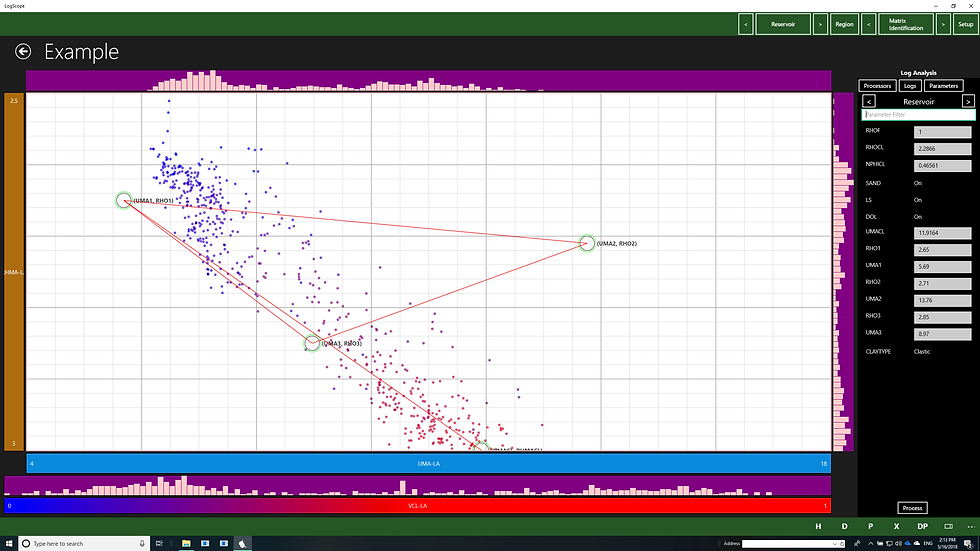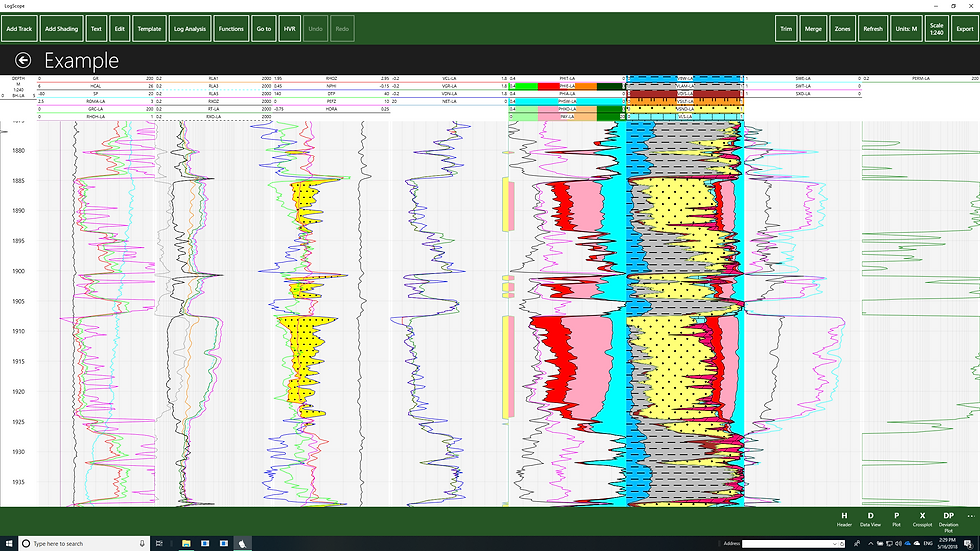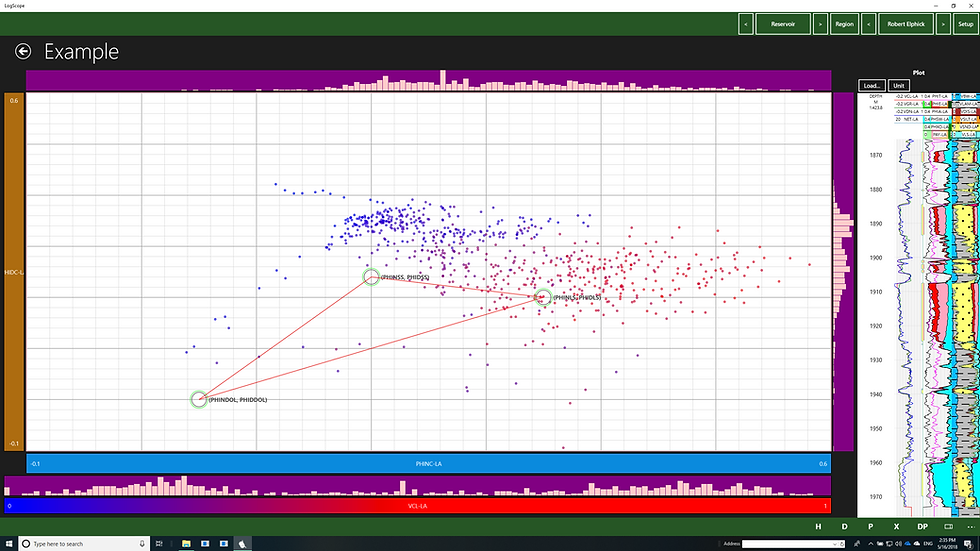top of page
Search
• Nicholas Harvey

# LOGSCOPE BASIC AND ADVANCED PETROPHYSICAL EVALUATION

Harvey Rock Physics has released the latest version of the LogScope software with the following capabilities:

• Project window complimented by map window

• Cross section capability added - can plot up to 533 wells

• Tadpole for formation dip and azimuth added to basic display plots

• Basic log analysis.

Basic Log Analysis

The basic evaluation in LogScope was intentionally designed and constructed to facilitate the analysis of logs to produce a simple evaluation that included Total and effective porosity as well as saturation, permeability and a lithologic model. The model would use the Neutron-Density cross-plot shown below to allow computation of porosity and Vclay from the neutron density.The primary lithology was determined by where the (NPHIMA, RHOMA) point was positioned. If it was positioned at 2.71 g/cc it was Limestone and if at 2.86 g/cc it was Dolomite. 2.65 g/cc as shown here would cause it to be sandstone.

If no photoelectric effect was included in the log suite run the analysis would consist of shale plus the primary lithology as shown below.If the photoelectric effect is included for the basic model the RHOMA versus UMA cross plot will be used to identify the proportion of of each lithology as shown below.This will yield the following analysis:The BASIC Log Analysis is limited to using the most common clay indicators which are the Gamma Ray and the Neutron Density and will take the minimum or the one selected.

Note there are cross plots such as M factor and N Factor cross plot amongst others that are inactive or lack the points that the advanced Petrophysical interpretation takes advantage of.

If a LogScope advanced petrophysical interpretation is sent you, you will be warned that your licensed LogScope version will not be able to process using the model used but you will be able to process using the basic model.

The models are interchangeable and a basic interpretation can be sent to a person with the advanced petrophysical module and vice-versa.

When contrasted with the Basic Log Analysis, LogScopes advanced log analysis provides a more extensive range of tools for interpretation. It builds on the BASIC model by adding the following:

• BASIC - Basic single matrix or PEF model

• DMM - Deterministic Mineral Model

• SSS - Silty Shaley Sand Model

• TWA - Two or Dual water model

• Geomechanical property computation

• TOC computation

• Sigma Processing

• NMR processing (produces Binned pore distribution, VCLAY and Porosity)

• Coal Bed Methane

If we take the example interpretation we were using before the same process will yield the following interpretations

The BASIC model will result in the following interpretation where the photo electric effect is included:If the photoelectric effect is excluded the plot will look like the following:Not much difference from the basic except the clays are broken down as dispersed and laminar.

If we change the model to the DMM model without the PEF the advanced will take advantage of the Robert-Elphick approach which is shown in the figure below.It uses the porosity calculated from density and porosity to resole out four components (fourth is heavy mineral) which is not plotted on here. LogScope has ability to plot log tracks next to cross plots as shown above. The interpretation is expanded below:If the PEF is switched back on it performs the computation in a similar manner to the BASIC model but calculates the clays.To trial these and any other features one can download Logscope from here